Neural network potential energy surface and dynamical isotope effects for the N+(3P) + H2 → NH+ + H reaction

Zijiang Yang a, Shufen Wang a, Jiuchuang Yuanb and Maodu Chen*a
aKey Laboratory of Materials Modification by Laser, Electron, and Ion Beams (Ministry of Education), School of Physics, Dalian University of Technology, Dalian 116024, P. R. China. E-mail: mdchen@dlut.edu.cn
bState Key Laboratory of Molecular Reaction Dynamics, Dalian Institute of Chemical Physics, Chinese Academy of Science, Dalian 116023, P. R. China

Received 17th May 2019 , Accepted 6th August 2019

First published on 7th August 2019

The N+(3P) + H2(X1Σ+g) → NH+(X2Π) + H(2S) reaction is important for initiating the chain reaction of ammonia synthesis in the universe. To study the dynamics of this reaction, a global accurate potential energy surface (PES) of the ground state NH+2 was constructed by combining numerous high-level ab initio energy points with the permutation invariant polynomial neural network method. Utilizing this newly constructed PES, time-dependent wave packet calculations were performed on the state-to-state reactions of N+(3P0) + H2 (v = 0, j = 0) and N+(3P0) + D2 (v = 0, j = 0) in order to study the microscopic reaction mechanisms and dynamical isotope effects. Isotope effects have a significant influence on the rovibrational state distributions and state resolved angle distributions of the product. The total differential cross sections (DCSs) present in the aforementioned reactions are dominated by both forward and backward scattering, as expected from the observable deep well along the reaction path. Meanwhile, the rovibrational state-resolved DCSs show that both reactions are not entirely statistical at the state-to-state level.

1 Introduction

The nitrogen atom and nitrogenous molecules are important tracers when studying interstellar objects; nitrogen hydrides or their ions are also of significance in astrophysics due to their abundance in the universe. One of the simplest nitrogen chemical reactions of N+(3P) + H2(X1Σ+g) → NH+(X2Π) + H(2S) has been suggested as the first step in the synthesis of ammonia within dense interstellar clouds.1,2 In addition, as a prototype for understanding ion–molecule reaction dynamics, it has highly interesting characteristics for study because of its near thermoneutrality. Especially, the dynamical behaviors are substantially different when the hydrogen undergoes isotopic substitution; numerous studies3–12 have focused on these isotope effects, which have been consecutively explored over the past 30 years.

A variety of experimental results for the title reaction and its isotopic counterparts, such as velocity-angle distributions13,14 and integral cross sections (ICSs),4,9 have been reported and provide abundant reference data for theoretical studies. Additionally, according to reported ab initio calculations,15 the title reaction processes a deep potential well (∼6.5 eV) path, resulting in the formation of long-lived complex NH+2 at low energies, and only a limited number of states are included due to its slight endothermicity. Thus, various statistical methods11,12,16,17 have been employed to treat the reactions of N+(3P) with H2 and its isotopic variants. Gerlich16 applied dynamically biased statistical theory to obtain the state-specific ICSs for the title reaction. The calculated results were very comparable with known experiments; however, large discrepancies occurred upon replacing H2 molecule with D2 molecule. Recently, Grozdanov et al.11 formulated a new statistical model to calculate the ICSs of reactions involving N+ with H2, D2 and HD molecules. The fine structure states of N+ ion were treated equally to other internal motions, and the calculated results for the three reactions could reproduce the experimental ICSs. In later work,12 the authors concluded that the dynamics results were strongly dependent on the fine structure state of N+ ion and the initial rotation state of the reactant H2 or HD.

However, theoretical treatment of statistical models is limited due to the addition of certain assumptions. A better method for accurately describing the characteristics of the title reaction is based on the precise potential energy surface (PES) that covers the entire reactant and product regions. The first ground state PES of NH+2 was constructed by Gittins and Hirst.18 The energy points were calculated via the configuration interaction (CI) method, and a deep 3B1 well at C2v symmetry was represented in the PES. However, a crucial issue was that their calculations of the product generated the wrong electronic state of NH+(a4Σ), which is actually the first excited state of NH+ molecule.19 Gonzalez et al.20 presented an ion-induced single surface model for the title reaction and discovered that the 3A2 well plays a predominant role in the energy range of 1 to 4 eV. The most recent PES for the NH+2 system was reported by Wilhelmsson et al.21 in 1992. In their work, a total of 752 configurations calculated by the complete active space self-consistent (CASSCF) and multi-reference configuration interaction (MRCI) methods were used to fit the analytical form, and the average least-squares deviation was 0.026 eV. Based on these PESs, dynamics simulations of the N+ and H2 reaction and its isotopic variants using classical trajectory calculations22 or the quasiclassical trajectory (QCT) method6,7,20,23 have been extensively implemented.

Although the QCT method or statistical models can describe the mechanism of the reactions of N+(3P) with H2 and its isotopic variants to some extent, the significant quantum effects are not included. The structure of the deep well and its nearly thermoneutral character imply that the quantum scattering resonances and the zero point energy (ZPE) effect are highly crucial to the reaction dynamics. Performing quantum wave packet calculations on the title reaction is extremely expensive due to the numerous quasi-bound states supposed by the deep well, which require a large number of grids and a long propagation time in order to achieve evolution of the wave packet. It is well known that the only quantum dynamics results for the N+(3P) + H2 reaction were calculated using the time-dependent wave packet (TDWP) method proposed by Russell and Manolopoulos.24 They performed TDWP calculations for the reactions of N+(3P) + H2 (v = 0 and 1, j = 0 and 1) on the Wilhelmsson PES. The ICS results of the ground vibrational reactions showed different behaviors compared with QCT calculations due to the inability of the traditional QCT method to treat the vibrational ZPE effect of the product molecule. In the QCT calculations, only the ZPE of the reactant H2 molecule was corrected; thus, the title reaction became exothermic.

According to previous studies, traditional QCT calculations are unable to correctly describe the dynamical behaviors of the title reaction. The ZPE problem can be solved to some extent using various corrective methods; however, the Gaussian–Binning method displays excellent corrective abilities toward this shortcoming of the QCT method. The Gaussian–Binning procedure25,26 has been successfully incorporated into several barrierless reactions, such as H+ + H227,28 and C(1D) + H229 reaction systems, and the calculated results were in good agreement with the quantum dynamics calculations. Another basic problem of the QCT method is its poor capability to describe quantum resonances, which are vital for deep well reactions. Therefore, using only quantum methods can give perfect dynamics information for the title reaction. Although quantum dynamics calculations for the title reaction have been reported, the inaccuracy of the results is attributed to the lack of reliable PES. The error of the Wilhelmsson PES is relatively large due to the limitations of early computational conditions. In addition, ab initio calculations30 and a recent experimental study10 have proved that the endothermicity of the reaction (33 meV) on the Wilhelmsson PES is too large. Hence, performing quantum dynamics calculations for the title reaction on a high-precision global PES is necessary to improve the accuracy of the dynamics results. Furthermore, quantum dynamics simulations of the N+(3P) + D2 reaction have not been presented to date, and it is desirable to perform these simulations to study the influence of isotope effects on the title reaction. Herein, a new accurate ground state PES of the NH+2 system is constructed using a large number of high level ab initio energy points and a neural network (NN) model. Based on our new PES, rigorous quantum TDWP calculations for the N+(3P0) + H2 (v = 0, j = 0) → NH+ + H and N+(3P0) + D2 (v = 0, j = 0) → ND+ + D reactions are implemented to study the reaction mechanism and the influence of isotope effects on the dynamics results.

2 Potential energy surface

2.1 Ab initio calculations

The electronic energies of the ground state (13A′′) NH+2 within Cs symmetry were calculated by the internally contracted MRCI method31,32 with the Davidson correction (icMRCI + Q), as implemented in the MOLPRO package.33 First, the Hartree–Fock wave functions were calculated as an initial guess, followed by CASSCF34,35 calculations for the seven equally weighted states (13A′′, 23A′′, 33A′′, 13A′, 11A′, 21A′, 11A′′). The icMRCI + Q calculations were carried out based on the optimized wave functions of the CASSCF process. In the CASSCF and icMRCI + Q calculations, six valence electrons are included in seven active orbitals (6a′ + 1a′′). The augmented correlation-consistent polarization valence quadruple-zeta (AVQZ) basis set36 and the Dunning-weighted correlation consistent polarized core-valence triple-zeta (WCVTZ)37 basis set were used for H and N atoms, respectively, which ensured accuracy of the calculations and convergence of key energy points by testing numerous geometries. A large number of ab initio energies over a great range of configuration spaces were chosen in the Jacobi coordinates, where the N+–H2 region was defined by 0.9 ≤ rHH/a0 ≤ 25, 0.8 ≤ RN+–HH/a0 ≤ 25, and 0 ≤ θ/degree ≤ 90 and the H–NH+ region was defined by 0.8 ≤ r+NH/a0 ≤ 28, 0 ≤ RH–NH+/a0 ≤ 25, and 0 ≤ θ/degree ≤ 180.

2.2 Fitting the potential energy surface

Representing global PESs from ab initio energies is challenging but can be achieved by combining electronic structure calculations with advanced machine learning technologies. Artificial NNs and Gaussian process regression are the most popular machine learning methods to fit PESs by a large number of ab initio data; these methods have been widely applied to numerous molecular systems.38–46 In this work, the NN method was used to obtain the analytical PES of the NH+2 system. The expression of the global surface consists of two-body and three-body terms and is written as follows: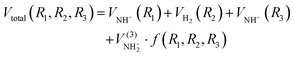(1)
where R1, R2 and R3 are the internuclear distances of NH+a, HH and NH+b, respectively. For the ion–molecule reaction, the accuracy of the long-range potential has substantial influence on the dynamics results; thus, a switch function f(R1, R2, R3) was used to better describe the PES in the long-range region, and it is written as follows: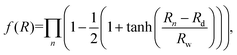(2)
where Rn represents the bond length of two atoms and Rd and Rw are the central position of f(R1, R2, R3) and the switch strength constant, respectively. In the process of two-body term fitting, the NN structure included two hidden layers, and 6 neurons were included in each hidden layer. 97 and 92 ab initio points were generated to obtain the potential energy curves of HH and NH+, and the root mean square errors (RMSE) are 0.10 and 0.12 meV, respectively. Fig. 1 shows the potential energy curves (PECs) of the H2(X1Σ+g) and NH+(X2Π) molecules, where the fitting of the PECs is in good agreement with the original ab initio data. The equilibrium bond length and dissociation energy of H2(X1Σ+g) on the fitted PECs are 1.402 Bohr and 4.71 eV, respectively, which compare well with the corresponding experimental data47 of Re = 1.401 Bohr and De = 4.74 eV. In the case of NH+(X2Π) molecule, the equilibrium bond length and dissociation energy are 2.047 Bohr and 4.64 eV, respectively, showing good agreement with the experimental results48 of Re = 2.043 Bohr and De = 4.65 ± 0.5 eV. Thus, the fitting results for the two-body potential are sufficiently accurate.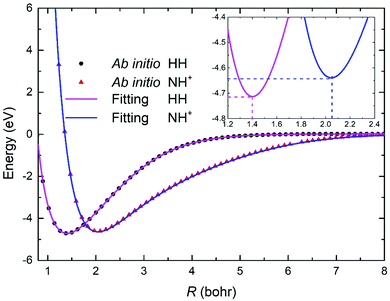Fig. 1 Comparison of ab initio data and fitting results for the potential energy curves of H2(X1Σ+g) and NH+(X2Π).

For the three-body term, permutation invariant polynomials (PIP)49,50 were employed to solve the problem of the adaptation of permutation symmetry caused by the two identical H atoms. The geometries after PIP preprocessing were used in the input layer of the NN. First, the bond length between two atoms was transformed as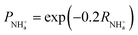(3)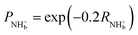(4)
 PHH = exp(−0.2RHH) (5)
Then, the following PIPs were adopted: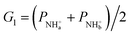(6)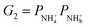(7)
 G3 = PHH (8)
Finally, G1, G2, and G3 were given normalized treatment as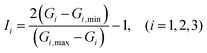(9)
where Gi,min and Gi,max are the minimum and maximum values of Gi, respectively. The NN included two hidden layers with 12 neurons, and the Levenberg–Marquardt algorithm51 was applied to train the NN. The final three-body NN expansion is presented as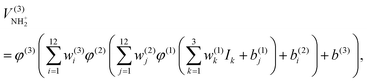(10)
where ω and b represent the corresponding weights and bias, which were optimized by training the NN. The linear and hyperbolic tangent functions were used as the transfer functions ϕ in the output layer and hidden layers, respectively. To avoid overfitting, 30519 energy points were divided randomly into three sets, namely the training set (90 percent), testing set (5 percent), and validation set (5 percent). The overall RMSE of the PIP-NN PES is only 2.7 meV.

2.3 Features of the potential energy surface

Fig. 2 shows the potential energies at four different insertion angles in the reactant Jacobi coordinates, where rHH and RN+–HH are the HH bond length and the distance between N+ and the center of mass of the H2 molecule, respectively, and θ is the angle between rHH and RN+–HH. The energies are relative to the N+ + H2 dissociation limit, and the maximum energy was set as 5 eV in order to clearly display the structures of the wells. There are two wells at every angle, which correspond to the 3B1 and 3A2 wells at the C2v structure, respectively. As the insertion angle decreases, the deeper well corresponding to the 3B1 state at the C2v structure becomes less accessible and the depth of the outer 3A2 well slowly decreases. The 3B1 well is located at rHH = 3.76 Bohr at the T-shaped symmetry, indicating the involvement of a bond stretching process prior to complex NH+2 formation. Moreover, there is a saddle point between the two wells, which can be attributed to prevention of the crossing of the two lowest electronic states.20 In the case of C2v symmetry, the complex NH+2 processes form at the deep 3B1 state well via the 3A23B1 intersection, and the two lowest states 3A2 and 3B1 return to the adiabatic ground state 3A′′ in the Cs geometry. In the present work, the ground state PES is constructed in the adiabatic representation, and the conical intersection is hampered by the limitation of the Born–Oppenheimer approximation. Thus, the origin of the repulsive regions is the interaction of the first excited state.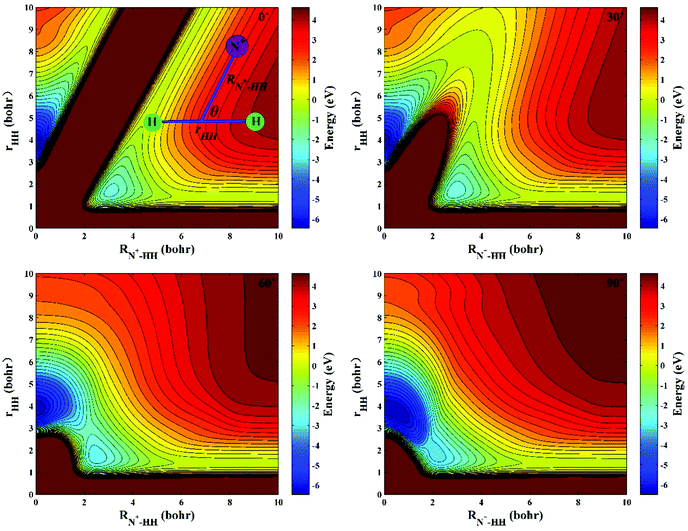Fig. 2 Potential energy surfaces of the ground state NH+2 for insertion approaches at four θ angles (0°, 30°, 60° and 90°).

The geometries and energies of the stationary points obtained on the new PES and original ab initio calculations are listed in Table 1 and compared with reported results. The energy values are relative to the N+(3P) + H2 dissociation limit. For the C2v geometry, the equilibrium structure corresponds with the 3B1 well, which is a highly crucial structure to the title reaction. As shown, the results calculated on the new PES are in good agreement with the original and previous ab initio calculations,15 and the Wilhelmsson PES underestimates the depth of the well. However, there is a large deviation between our data and previous ab initio calculations52 for the minimal energy of the collinear structure. This may be because the electron correlation energy cannot be treated well using the self-consistent field method with rough CI corrections. The new PES can better reproduce the high-level ab initio calculations at the stationary points, suggesting enhanced reliability in reaction dynamics studies.

Table 1 Stationary points of the ground state NH+2
C 2v r HH (Bohr) R N+–HH (Bohr) Energy (eV)
NN PES 3.76 0.48 −6.49
Wilhelmsson PES21 3.66 0.64 −6.15
icMRCI + Q 3.76 0.48 −6.50
MRD-CI15 3.67 0.49 −6.45

C ∞v R HH (Bohr) R +NH (Bohr) Energy (eV)
NN PES 1.68 2.24 −2.20
Wilhelmsson PES21 1.70 2.27 −2.05
icMRCI + Q 1.67 2.24 −2.22
SCF-CI52 1.60 2.50 −1.76

The contour maps of the new PES at four different N+–H–H angles (45°, 90°, 135° and 180°) are presented in Fig. 3. RHH represents the bond length of the two H atoms, R+NH is the distance between the N+ and one of the H atoms, and the N+–H–H angle is the included angle between RHH and R+NH. The energy is set as zero at the N+ + H2 dissociation limit. Two valleys exist at the bottom and left, corresponding to the N+(3P) + H2(X1Σ+g) channel and NH+(X2Π) + H(2S) channel, respectively. The depth of the two valleys is comparable, indicating that the title reaction is approximately thermoneutral. The deep well structure connects the two valleys at all four approaching angles, highlighting the important role of quantum resonances in the reaction dynamics. At the beginning of the title reaction, the N+ ion and H2 molecule convene in the bottom valley, generating the long-lived NH+2 complex in the deep well and eventually dissociating to the NH+ molecule and H atom in the left valley. To more clearly describe the characteristics of the title reaction on the new PES, the minimum energy paths (MEPs) of the N+(3P) + H2(X1Σ+g) → NH+(X2Π) + H(2S) reaction at four N+–H–H angles (45°, 90°, 135°, 180°) are shown in Fig. 4. The MEPs were determined by scanning the PES, as shown in Fig. 3, at different coordinates (RHHR+NH) to obtain the minimum values of the potential energy. The depth of the well decreases with increasing S–H–H angle, suggesting that the NH+2 complex has a shorter lifetime when the reaction proceeds at a large approaching angle. It can be seen from the minimum energy paths that the title reaction is weekly endothermic, with an endothermicity of approximately 83 meV; this is consistent with the ab initio calculations. Table 2 lists the molecular constants of the reactants and products, which were obtained from the analytical PES. When the ZPEs of the reactants and products are considered, the endothermicities of the N+ + H2 and N+ + D2 reactions are approximately 8 and 23 meV, respectively, which is in good agreement with the experimental measurements8 of 11 ± 3 and 29 ± 3 meV.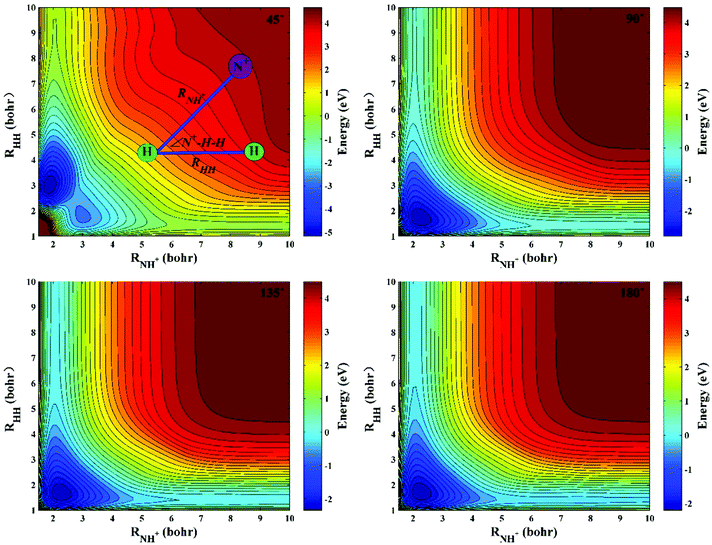Fig. 3 Potential energy surfaces of the ground state NH+2 at four N+–H–H angles (45°, 90°, 135° and 180°).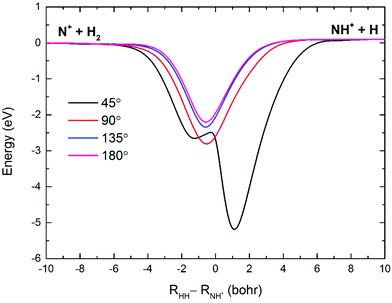Fig. 4 Minimum energy paths for the N+(3P) + H2(X1Σ+g) → NH+(X2Π) + H(2S) reaction at four N+–H–H angles (45°, 90°, 135° and 180°).
Table 2 Molecular constants of the reactants and products
ω e (cm−1) ω e x e (cm−1) D 0 (eV) ZPEa (eV)
a ZPE determined by ωe/2 − ωexe/4.
H2(X1Σ+g) 4398.2 121.3 4.445 0.269
D2(X1Σ+g) 3109.1 60.2 4.523 0.190
NH+(X2Π) 2870.3 66.5 4.463 0.176
ND+(X2Π) 2062.1 38.8 4.512 0.127

Fig. 5(a) displays the potential energy of N+ ion moving around the H2 molecule with a fixed bond length of H2 (1.401 Bohr); the energy is set as zero when the N+ ion is far from the H2 molecule. A 2.67 eV deep well is present at x = 0.0 Bohr and y = 2.3 Bohr. The depth of the well increases as the HH bond stretches, which can promote insertion collisions at low energies as the N+ ion slowly approaches the H2 molecule. Similar contours to Fig. 5(a) for a H atom moving around the NH+ molecule are presented in Fig. 5(b). The NH+ bond length is set to the equilibrium distance (2.043 Bohr), and the energy is set as zero when the H atom is far from the NH+ molecule. It can be seen that a deeper well exists around the N+ ion, implying that the H atom can more readily escape from the side of the H in the NH+ molecule in the title reaction.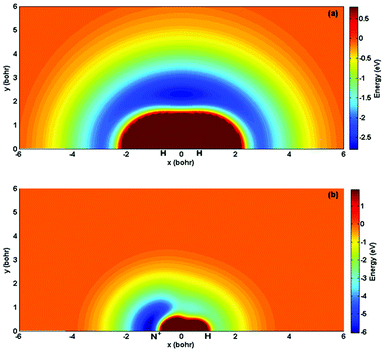Fig. 5 (a) Contour plot of the PES for the N+ ion moving around the H2 molecule fixed at the equilibrium distance (1.401 Bohr). (b) Contour plot of the PES for a H atom moving around the NH+ molecule fixed at the equilibrium distance (2.043 Bohr).

3 Dynamics calculations

The TDWP method is a powerful tool for calculating atom–diatom reactive scattering and can give accurate information about the state-to-state reaction dynamics. Only an abbreviated outline is introduced here; more detailed descriptions of the method can be found in previous literature reports.53,54 In the body fixed representation, the Hamiltonian is expressed as follows: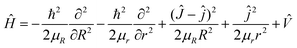(11)
where R is the distance from the N+ ion to the center of mass of the H2 molecule and r is the bond length of the H2 molecule. μR and μr are the corresponding reduced masses associated with the R and r coordinates. Ĵ and ĵ are the total angular momentum operator of the NH+2 system and the rotational angular momentum operator of the H2 molecule, respectively.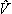is the potential energy of the NH+2 system which is obtained on the NN PES. The state-to-state S-matrix was extracted by the reactant coordinate-based method,55,56 and the second-order split operator was adopted to evaluate the wave function. The state-to-state reaction probability was calculated by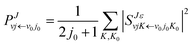(12)
The state-resolved ICSs were obtained by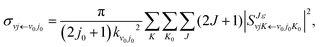(13)
where kv0j0 is the momenta in the entrance channel. The state-resolved differential cross sections (DCSs) can be calculated by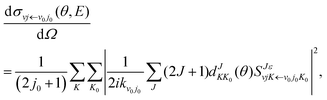(14)
in which θ is the scattering angle and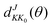represents the element of the reduced Wigner rotation matrix.

The initial rovibrational states of the reactant H2 molecule were set as v0 = 0 and j0 = 0, and the TDWP calculations included all Coriolis couplings. All partial waves were calculated up to J = 75 for the N + H2 reaction and J = 81 for the N + D2 reaction, yielding converged ICSs and DCSs for collision energies beyond 0.7 eV, which was set as the upper limit of the collision energy in the reaction dynamics. Large grids and sufficient propagation times were used to ensure that the wave packet could reach the low energy region. The optimal numerical parameters used in the calculations are listed in Table 3 by numerous convergence tests.

Table 3 Numerical parameters used in the TDWP calculations
N+(3P) + H2 N+(3P) + D2
Grid ranges and sizes R (Bohr) ∈ [0.1, 22.0], NtotR = 389 R (Bohr) ∈ [0.1, 22.0], NtotR = 389
r (Bohr) ∈ [0.4, 16.0], Ntotr = 299 r (Bohr) ∈ [0.4, 16.0], Ntotr = 299
N j = 100 N j = 100
Initial wave packet R c = 16.0 Bohr R c = 16.0 Bohr
Δ R = 0.20 Bohr Δ R = 0.18 Bohr
k 0 = (2E0μR)1/2 with E0 = 0.50 eV E 0 = 0.50 eV
Propagation time 100000 a.u. 100000 a.u.
Highest J value 74 81

The collision energy dependence of the total reaction probabilities for the N+ + H2 and N+ + D2 reactions with four different partial waves are presented in Fig. 6. An extremely dense oscillation feature was found in the reaction probability curves, which can be attributed to the formation of long-lived complexes in the deep well. The domination of long-lived resonance is similar to the typical insertion reactions of S(1D) + H257 and C(1D) + H2,58 and the title reaction has a sharper oscillation peak because of the deeper well. For J = 0, the threshold of the two reactions is consistent with the corresponding endothermicity, implying a barrierless path at low collision energies. With increasing J, the oscillation amplitude becomes less pronounced due to the increased centrifugal barrier. In the case of high J values, the threshold of the N+ + H2 reaction is larger than that of the N+ + D2 reaction due to the heavier reduced mass of ND+2. Because the increased collision energy diminishes the constraint of the deep well, the resonance peak becomes broader at high energy.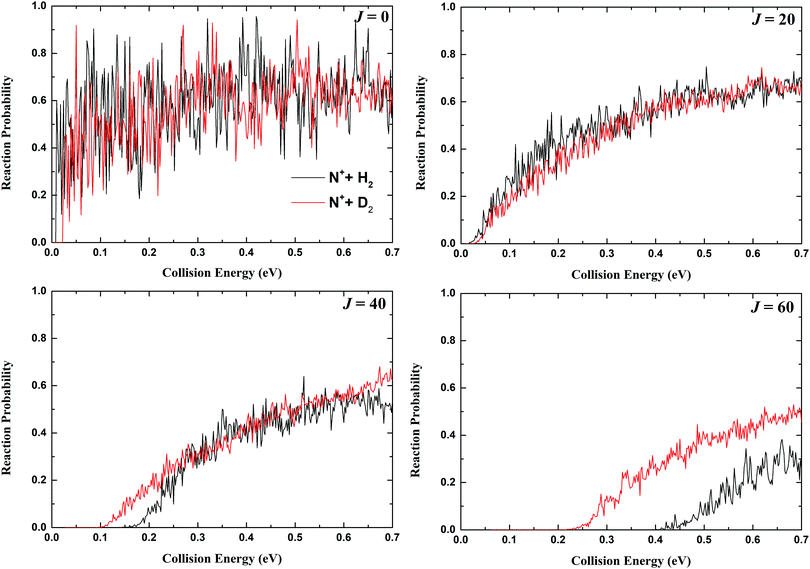Fig. 6 Total reaction probabilities for the N+(3P) + H2 and N+(3P) + D2 reactions as a function of collision energy with four different total angular momentums (J = 0, 20, 40, 60).

The total ICSs for the N+(3P) + H2 and N+(3P) + D2 reactions obtained from the TDWP calculations on the NN PES together with the experimental measurements9 using a guided ion bean mass spectrometer and calculated by statistical theory11 are shown in Fig. 7. Previous TDWP calculations24 for the N+(3P) + H2 reaction on the Wilhelmsson PES are also presented. The ICS values for the N+(3P) + H2 reaction calculated by the TDWP method on the NN PES are larger than those on the Wilhelmsson PES due to the smaller reaction threshold of the NN PES. As shown, the quantum resonances remain strong on the TDWP ICS curves even though various partial waves have been summed, and the oscillatory structures calculated on the NN PES are narrower than the previous results due to the deeper well of the NN PES. The TDWP results show that the total ICS increases slightly as a function of collision energy and then nearly reaches a plateau, which is characteristic of an endothermic reaction.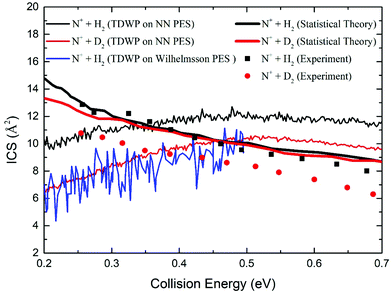Fig. 7 Total ICSs for the N+(3P) + H2 and N+(3P) + D2 reactions as a function of collision energy compared with previous TDWP calculations on the Wilhelmsson PES, the results calculated by statistical theory and available experimental values.

In general, the ICS values generated by the TDWP calculations are close to the results calculated by the statistical method and the experimental data. However, the collision energy dependence of the ICSs obtained by the TDWP calculations is in contrast with the statistical and experimental results, which present a monotonic decreasing dependence of collision energy. This arises because the statistical and experimental data include the excited spin–orbit states of N+ ion and the higher rotational states of the H2 or D2 molecule. In the previous experiment, the rotational temperature of H2 or D2 was 105 K, and the N+ ion was produced in a thermal distribution at 300 K. In this case, the rotational state populations of H2 at j = 0, 1, 2, and 3 were 0.243, 0.749, 0.008 and 0.0004, respectively, and the rotational state populations of D2 at j = 0, 1, 2, 3, and 4 were 0.472, 0.322, 0.193, 0.012 and 0.001, respectively. The spin–orbit state distributions for N+ ion at 3P0, 3P1 and 3P2 were 0.17, 0.39 and 0.44, respectively. In the statistical theory results, the spin–orbit states of N+ ion were treated equally to the vibrational and rotational states of H2 and D2, and the endoergicities of the N+(3P) + H2 and N+(3P) + D2 reactions were set as 18.45 and 46.32 meV, respectively. The electronic energy of N+ ion is 16.2 meV for the fine structure state 3P2, and the rotational energy of H2 molecule at j = 1 is 14.4 meV, which are larger than the endoergicity of the title reaction. The averaging effects of the excited spin–orbit states and higher rotational states allow the two reactions to become exothermic. It is clear that the statistical theory results successfully reproduce the experimental data of the N+(3P) + H2 reaction even when some assumptions are included at the energy range. However, in the case of the N+(3P) + D2 reaction, the statistical theory results and experimental data have relatively large deviations. In addition, the statistical method cannot characterize the quantum resonances, which is crucial for a deep well reaction. Herein, only rigorous quantum dynamics calculations on the ground adiabatic PES for the state-resolved reactions of N+(3P0) + H2 and N+(3P0) + D2 were performed; thus, they displayed different dynamics behavior compared with the statistical and experimental results.

The rovibrational state-resolved ICSs of the product NH+ and ND+ molecules at four collision energies (0.1 eV, 0.3 eV, 0.5 eV and 0.7 eV) calculated by the TDWP method are shown in Fig. 8. At lower collision energies, only the ground vibrational state is populated, and the v′ = 1 and v′ = 2 channels are opened with increasing collision energy. As shown, the products are difficult to excite to higher vibrational states, implying that the energy conversion efficiency is low. The main reason for this is the presence of a deep well on the reaction path: the N+ ion collides with the H2 or D2 molecule, generating the long-lived complex. One H or D atom is ejected only when the rotational quantum number of the continuously rotating complex is large enough to cleave the HH or DD bond. Thus, the majority of the collision energies are transformed into translational energy of the product molecules, and the transformed internal energy of the products is relatively low. In addition, a part of the collision energy is used to overcome the centrifugal barrier at the exit channel. As the collision energy increases, more rotational states are populated, and the peak of the rotational state distribution shifts to a higher j′ value; this indicates that more energy is transformed into the rotational energy of the NH+ or ND+ molecule. Compared with the N+(3P) + H2 reaction, the N+(3P) + D2 reaction more easily excites the product to high rovibrational states even though it has a larger threshold. This is because the vibrational frequency and rotational constant of ND+ molecule are smaller than those of NH+ molecule, and the title reaction is very sensitive toward this subtle difference. Moreover, the N+(3P) + D2 reaction has smaller centrifugal barriers than the N+(3P) + H2 reaction at the special partial wave, which leads to the transformation of more collision energy into internal energy. Therefore, the isotope effects have considerable influence on the product rovibrational state distributions.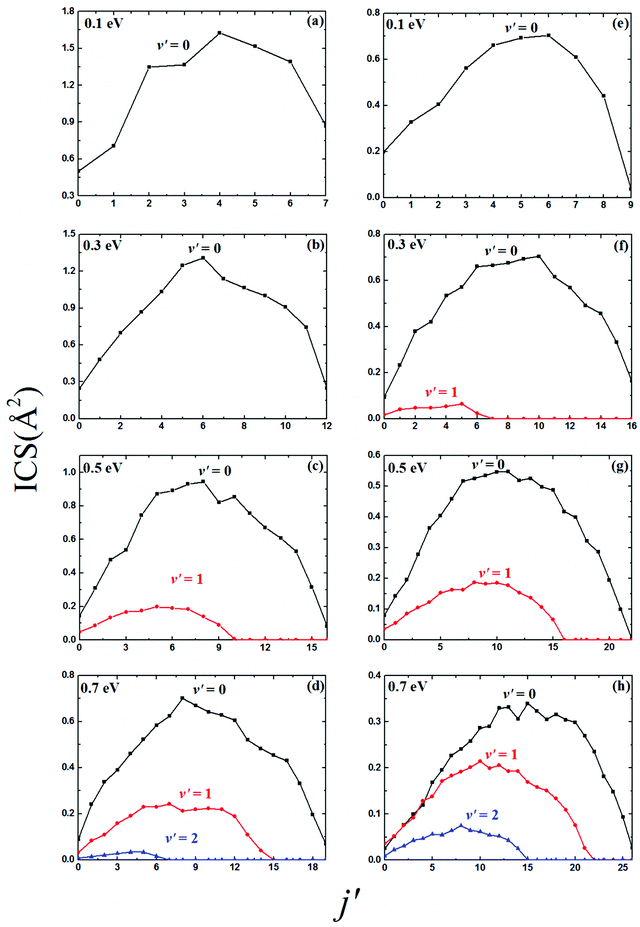Fig. 8 Rovibrational state-resolved ICSs for the (a–d) N+(3P) + H2 and (e–h) N+(3P) + D2 reactions at four collision energies (0.1, 0.3, 0.5 and 0.7 eV).

The DCSs can describe scattering features in greater detail, whereas the angular distributions of the NH+ and ND+ molecules formed by the two reactions have not been presented to date. The three-dimensional plots of the total DCSs for the N+(3P) + H2 and N+(3P) + D2 reactions at collision energies below 0.7 eV are given in Fig. 9. The angular distributions of NH+ and ND+ molecules are nearly forward-backward symmetric in the whole selected collision energy range. The peaks appear at two extreme angles, which is attributed to the long-lived complex-forming mechanism. The collision energy dependence of the DCSs shows sharp, dense oscillatory structures at each scattering angle; this stems from the long-lived quasi-bound states supported by the deep well, which lead to densely distributed quantum resonances.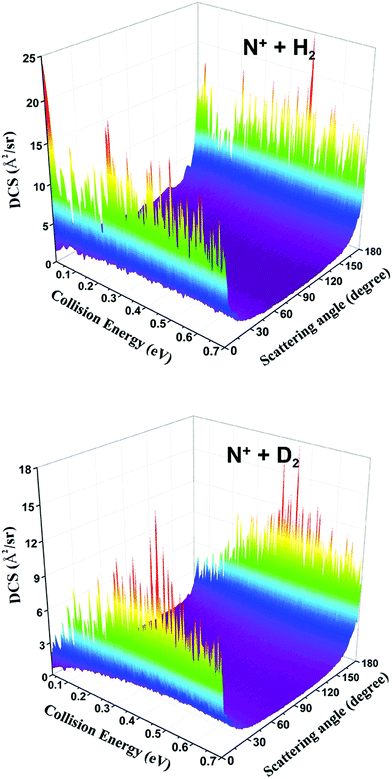Fig. 9 Three-dimensional plots of the total DCSs for the N+(3P) + H2 and N+(3P) + D2 reactions as a function of collision energy.

Fig. 10 displays the total and vibrational state-resolved DCSs of the two reactions at 0.7 eV collision energy. The total DCSs show that the forward and backward peaks are almost equal, and the angle distributions are symmetric with respect to 90°. However, the symmetries of the DCSs are broken at the vibrational state-resolved levels of the product molecules for the two reactions. The forward scattering becomes more obvious when the product is excited to higher vibrational states, which suggests that both reactions demonstrate nonstatistical behavior at the vibrational state-resolved levels of the product molecules despite their complex-forming natures. Fig. 11 shows several rational states (j′ = 0, 5, 10, 15) of the resolved DCSs for the N+(3P) + H2 and N+(3P) + D2 reactions at 0.7 eV collision energy, and the corresponding vibrational state is v′ = 0. It is clear that the asymmetry of the forward–backward scattering is more apparent. For example, at the rotational state j′ = 10, the forward peak of the N+(3P) + H2 reaction is three times higher than the backward peak. In the case of the N+(3P) + D2 reaction, the peak at 0° disappears in the j′ = 0 state, and the forward bias becomes dominant when the product is excited to high rotational states. This asymmetric behavior is also found in some long-lived complex-forming reactions, such as the H+ + D2,27 S(1D) + H259 and H + O260 reactions. The basic reason for the asymmetric angle distributions may be the strong scattering resonances even after summation over all the partial waves. Therefore, the quenching effect of the quantum interferences between various partial waves is less efficient than intuitively expected at the state-resolved level. A comparison study59 of quantum and statistical methods for several typical complex-forming reactions has proved that statistical theories are unable to accurately study product state-resolved reaction dynamics, and the N+(3P) + H2 and N+(3P) + D2 reactions have similar nonstatistical behaviors. In addition, the DCSs of the two reactions have dramatic differences at specific rotation states; thus, the isotope effects have a significant impact on the state-resolved angle distributions of the product molecules.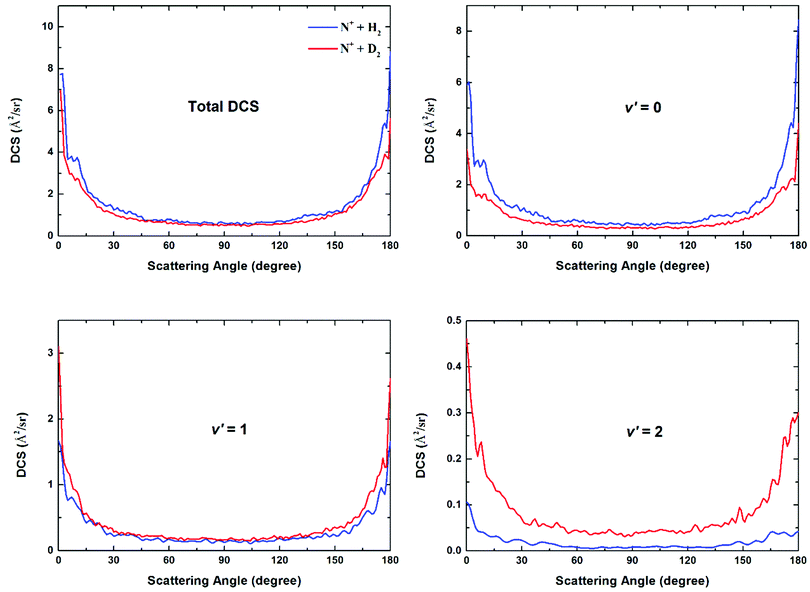Fig. 10 Total and vibrational state-resolved DCSs for the N+(3P) + H2 and N+(3P) + D2 reactions at 0.7 eV collision energy.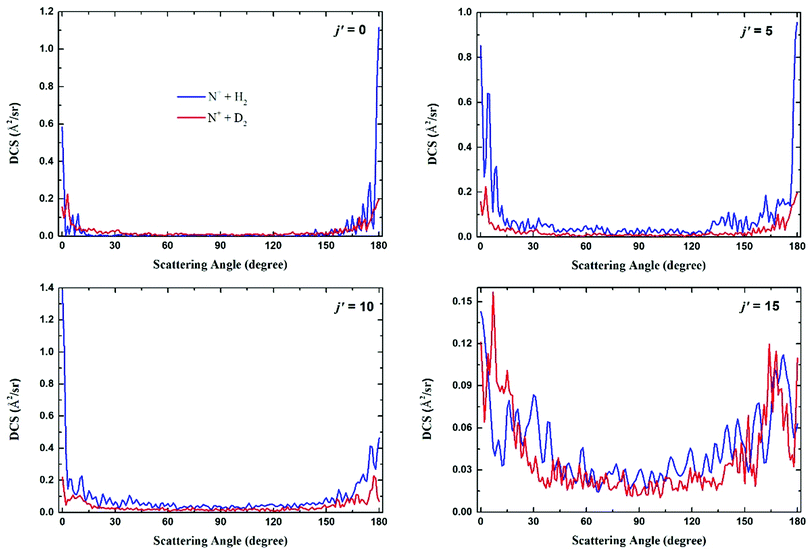Fig. 11 Several rotational state-resolved (j′ = 0, 5, 10 and 15) DCSs for the N+(3P) + H2 and N+(3P) + D2 reactions at v′ = 0 and a collision energy of 0.7 eV.

4 Conclusion

In the present work, a new PES for the ground state NH+2 system was constructed over a large coordinate space; it can be used to obtain accurate dynamics information for the significant interstellar reaction of N+(3P) + H2(X1Σ+g) → NH+(X2Π) + H(2S). The ab initio energy points are calculated using the icMRCI + Q method with high level basis sets (AVQZ for H atom and WCVQZ for N atom). A total of 30519 geometries were selected to fit the PES using the PIP-NN model, and the fitting RMSE was only 2.7 meV. The structures and characteristics of the new PES are described in detail and can give more accurate information than previous PESs. TDWP calculations for the N+(3P0) + H2 (v = 0, j = 0) and N+(3P0) + D2 (v = 0, j = 0) reactions were carried out on the NN PES to study the reaction mechanism and dynamical isotope effects. Obvious quantum resonances are found in the reaction probabilities, ICSs, and DCS curves due to long-lived complex formation in the deep well (6.49 eV). The rovibrational state-resolved ICSs reflect that the isotope effects greatly affect the state distributions of the product molecules. The total DCSs have almost forward–backward symmetric angular distributions; however, the state-resolved DCSs show nonstatistical behavior.

Herein, accurate quantum dynamics calculations for the N+(3P0) + H2 (v = 0, j = 0) and N+(3P0) + D2 (v = 0, j = 0) reactions were performed on the ground state adiabatic PES. A rigorous comparison of the theoretical results with experimental data is very difficult because it requires the inclusion of all accessible spin–orbit states of N+ ion and rotational states of H2 or D2 molecule. In addition, a set of accurate diabatic PESs correlated with the spin–orbit interaction should be used to calculate higher fine structure state reactions, which would be a very interesting but challenging task in future work.

Conflicts of interest

There are no conflicts to declare.

Acknowledgements

This work was supported by the National Natural Science Foundation of China (No. 11774043) and China Postdoctoral Science Foundation (No. 2018M631828).

References

1. A. Dalgarno, E. Herbst, S. Novick and W. Klemperer, Astrophys. J., 1973, 183, L131–L133 CrossRef CAS.
2. A. Dalgarno and J. H. Black, Rep. Prog. Phys., 1976, 39, 573–612 CrossRef CAS.
3. N. G. Adams and D. Smith, Chem. Phys. Lett., 1985, 117, 67–70 CrossRef CAS.
4. K. M. Ervin and P. B. Armentrout, J. Chem. Phys., 1987, 86, 2659–2673 CrossRef CAS.
5. J. B. Marquette, C. Rebrion and B. R. Rowe, J. Chem. Phys., 1988, 89, 2041–2047 CrossRef CAS.
6. M. Gonzalez, A. Aguilar and R. Sayos, Chem. Phys., 1989, 132, 137–151 CrossRef CAS.
7. G. Nyman and U. Wilhelmsson, J. Chem. Phys., 1992, 96, 5198–5212 CrossRef CAS.
8. P. Tosi, O. Dmitriev, D. Bassi, O. Wick and D. Gerlich, J. Chem. Phys., 1994, 100, 4300–4307 CrossRef CAS.
9. L. S. Sunderlin and P. B. Armentrout, J. Chem. Phys., 1994, 100, 5639–5645 CrossRef CAS.
10. I. Zymak, M. Hejduk, D. Mulin, R. Plasil, J. Glosik and D. Gerlich, Astrophys. J., 2013, 768, 86 CrossRef.
11. T. P. Grozdanov and R. McCarroll, J. Phys. Chem. A, 2015, 119, 5988–5994 CrossRef CAS PubMed.
12. T. P. Grozdanov, R. McCarroll and E. Roueff, Astron. Astrophys., 2016, 589, 735–745 CrossRef.
13. S. G. Hansen, J. M. Farrar and B. H. Mahan, J. Chem. Phys., 1980, 73, 3750–3762 CrossRef CAS.
14. B. H. Mahan and W. E. W. Ruska, J. Chem. Phys., 1976, 65, 5044–5051 CrossRef CAS.
15. S. D. Peyerimhoff and R. J. Buenker, Chem. Phys., 1979, 42, 167–176 CrossRef CAS.
16. D. Gerlich, J. Chem. Phys., 1989, 90, 3574–3581 CrossRef CAS.
17. G. Nyman, J. Chem. Phys., 1992, 96, 3603–3612 CrossRef CAS.
18. M. A. Gittins and D. M. Hirst, Chem. Phys. Lett., 1975, 35, 534–536 CrossRef CAS.
19. H. P. D. Liu and G. Verhaegen, J. Chem. Phys., 1970, 53, 735–745 CrossRef CAS.
20. M. Gonzalez, A. Aguilar and Y. Fernandez, Chem. Phys., 1986, 104, 57–66 CrossRef CAS.
21. U. Wilhelmsson, P. E. M. Siegbahn and R. Schinke, J. Chem. Phys., 1992, 96, 8202–8211 CrossRef CAS.
22. M. A. Gittins and D. M. Hirst, Chem. Phys. Lett., 1979, 65, 507–510 CrossRef CAS.
23. U. Wilhelmsson and G. Nyman, J. Chem. Phys., 1992, 96, 1886–1895 CrossRef CAS.
24. C. L. Russell and D. E. Manolopoulos, J. Chem. Phys., 1999, 110, 177–187 CrossRef CAS.
25. L. Bonnet and J. C. Rayez, Chem. Phys. Lett., 1997, 277, 183–190 CrossRef CAS.
26. L. Bonnet and J. C. Rayez, Chem. Phys. Lett., 2004, 397, 106–109 CrossRef CAS.
27. P. G. Jambrina, F. J. Aoiz, N. Bulut, S. C. Smith, G. G. Balint-Kurti and M. Hankel, Phys. Chem. Chem. Phys., 2010, 12, 1102–1115 RSC.
28. P. G. Jambrina, J. M. Alvarino, F. J. Aoiz, V. J. Herrero and V. Saez-Rabanos, Phys. Chem. Chem. Phys., 2010, 12, 12591–12603 RSC.
29. L. Banares, F. J. Aoiz, P. Honvault, B. Bussery-Honvault and J. M. Launay, J. Chem. Phys., 2003, 118, 565–568 CrossRef CAS.
30. R. Tarroni, P. Palmieri, A. Mitrushenkov, P. Tosi and D. Bassi, J. Chem. Phys., 1997, 106, 10265–10272 CrossRef CAS.
31. H. J. Werner and P. J. Knowles, J. Chem. Phys., 1988, 89, 5803–5814 CrossRef CAS.
32. P. J. Knowles and H. J. Werner, Chem. Phys. Lett., 1988, 145, 514–522 CrossRef CAS.
33. H. J. Werner, P. J. Knowles, G. Knizia, F. R. Manby and M. Schutz, Wiley Interdiscip. Rev.: Comput. Mol. Sci., 2012, 2, 242–253 CAS.
34. H. J. Werner and P. J. Knowles, J. Chem. Phys., 1985, 82, 5053–5063 CrossRef CAS.
35. P. J. Knowles and H. J. Werner, Chem. Phys. Lett., 1985, 115, 259–267 CrossRef CAS.
36. R. A. Kendall, T. H. Dunning and R. J. Harrison, J. Chem. Phys., 1992, 96, 6796–6806 CrossRef CAS.
37. D. E. Woon and T. H. Dunning, J. Chem. Phys., 1995, 103, 4572–4585 CrossRef CAS.
38. J. C. Yuan, D. He and M. D. Chen, Phys. Chem. Chem. Phys., 2015, 17, 11732–11739 RSC.
39. J. C. Yuan, D. He, S. F. Wang, M. D. Chen and K. L. Han, Phys. Chem. Chem. Phys., 2018, 20, 6638–6647 RSC.
40. Z. J. Yang, J. C. Yuan, S. F. Wang and M. D. Chen, RSC Adv., 2018, 8, 22823–22834 RSC.
41. J. Cui and R. V. Krems, Phys. Rev. Lett., 2015, 115, 073202 CrossRef PubMed.
42. J. Cui and R. V. Krems, J. Phys. B: At., Mol. Opt. Phys., 2016, 49, 224001 CrossRef.
43. B. Kolb, P. Marshall, B. Zhao, B. Jiang and H. Guo, J. Phys. Chem. A, 2017, 121, 2552–2557 CrossRef CAS PubMed.
44. Y. F. Guan, S. Yang and D. H. Zhang, Mol. Phys., 2018, 116, 823–834 CrossRef CAS.
45. B. Jiang, J. Li and H. Guo, Int. Rev. Phys. Chem., 2016, 35, 479–506 Search PubMed.
46. S. F. Wang, J. C. Yuan, H. X. Li and M. D. Chen, Phys. Chem. Chem. Phys., 2017, 19, 19873–19880 RSC.
47. K. P. Huber and G. Herzberf, Constants of Diatomic Molecules, Springer, 1979 Search PubMed.
48. R. Colin and A. E. Douglas, Can. J. Phys., 1968, 46, 61 CrossRef CAS.
49. B. J. Braams and J. M. Bowman, Int. Rev. Phys. Chem., 2009, 28, 577–606 Search PubMed.
50. B. Jiang and H. Guo, J. Chem. Phys., 2013, 139, 054112 CrossRef PubMed.
51. M. T. Hagan and M. B. Menhaj, IEEE Trans. Neural Netw. Learn. Syst., 1994, 5, 989–993 CrossRef CAS PubMed.
52. D. M. Hirst, Mol. Phys., 1978, 35, 1559–1568 CrossRef CAS.
53. Z. G. Sun, S. Y. Lee, H. Guo and D. H. Zhang, J. Chem. Phys., 2009, 130, 174102 CrossRef PubMed.
54. Z. G. Sun, H. Guo and D. H. Zhang, J. Chem. Phys., 2010, 132, 084112 CrossRef PubMed.
55. Z. G. Sun, X. Lin, S. Y. Lee and D. H. Zhang, J. Phys. Chem. A, 2009, 113, 4145–4154 CrossRef CAS PubMed.
56. S. Gomez-Carrasco and O. Roncero, J. Chem. Phys., 2006, 125, 054102 CrossRef PubMed.
57. J. C. Yuan, D. He and M. D. Chen, Sci. Rep., 2015, 5, 14594 CrossRef CAS PubMed.
58. Z. P. Sun, C. F. Zhang, S. Y. Lin, Y. J. Zheng, Q. T. Meng and W. S. Bian, J. Chem. Phys., 2013, 139, 014306 CrossRef PubMed.
59. P. Larregaray and L. Bonnet, J. Chem. Phys., 2015, 143, 144113 CrossRef PubMed.
60. Z. G. Sun, D. H. Zhang, C. X. Xu, S. L. Zhou, D. Q. Xie, G. Lendvay, S. Y. Lee, Y. Lin and H. Guo, J. Am. Chem. Soc., 2008, 130, 14962 CrossRef CAS PubMed.
 This journal is © the Owner Societies 2019